posts
rstats
python
Published

August 30, 2023

# Introduction

In this blog post, we talk about our experience teaching R and Python for geocomputation. The focus of the blog post is on geographic vector data, meaning points, lines, polygons (and their ‘multi’ variants) and the attributes associated with them. Geographic data analysis is a broad topic and in a later post we will cover raster data, meaning gridded data such as satellite images.

The context of this blog post is the OpenGeoHub Summer School 2023 which has courses on R, Python and Julia. The size and the diversity of the event has grown over the years. Noting that many events focus on just one language, and the advantages of diversity of languages and approaches, we wanted to follow-up in a blog post that could be useful to others.

OpenGeoHub 2023 was also a unique chance for the authors of the in-progress open source book, Geocomputation with Python to meet in person: the first time we have all been in one place at the same time.

The post is based on the following lecture notes, which we recommend checking out for deeper dives into the R and Python implementations of geocomputation:

# Comparing R and Python for vector geographic data analysis

We will start by loading core packages for working with geographic vector and attribute data. See detailed description of R and Python implementations in the respective lecture note sections.

``````import pandas as pd
from shapely import Point
import geopandas as gpd``````
``````library(sf)
library(tidyverse)
library(tmap)``````

## Creating geographic data

The following commands create geographic datasets ‘from scratch’ representing coordinates of a the faculty where the Summer School takes place, and few hotels, in Poznan, Poland. Most projects start with pre-generated data, but it’s useful to create datasets to understand data structures.

``````poi = gpd.GeoDataFrame([
{"name": "Faculty",        "geometry": Point(16.9418, 52.4643)},
{"name": "Hotel ForZa",    "geometry": Point(16.9474, 52.4436)},
{"name": "Hotel Lechicka", "geometry": Point(16.9308, 52.4437)},
{"name": "FairPlayce",     "geometry": Point(16.9497, 52.4604)},
], crs=4326)``````
``````poi_df = tribble(
~name, ~lon, ~lat,
"Faculty",        16.9418, 52.4643,
"Hotel ForZa",    16.9474, 52.4436,
"Hotel Lechicka", 16.9308, 52.4437,
"FairPlayce",     16.9497, 52.4604
)
poi_sf = sf::st_as_sf(poi_df, coords = c("lon", "lat"), crs = "EPSG:4326")``````

``````import urllib.request
import zipfile
import os
f = os.path.basename(u)
if not os.path.exists("data"):
urllib.request.urlretrieve(u, f)``````
``````u = "https://github.com/Robinlovelace/opengeohub2023/releases/download/data/data.zip"
f = basename(u)
if (!dir.exists("data")) {
}``````

Note that we can read directly from ZIP files with R and Python thanks to the GDAL Virtual File System.

## Unzipping data

We can unzip the data in R and Python: it contains spatial information about bus stops.

``````with zipfile.ZipFile(f, 'r') as zip_ref:
zip_ref.extractall()``````
``unzip(f)``

## Reading and printing geographic data

As shown below, Python and R implemenations to import a shapefile are similar. Note: we recommend using open file formats such as GeoPackage (`.gpkg`), as outlined in Geocomputation with R and Geocomputation with Python.

``````pol_all = gpd.read_file("zip://data.zip!data/osm/gis_osm_transport_a_free_1.shp")
pol_all``````
``````         osm_id  ...                                           geometry
0      27923283  ...  POLYGON ((16.84088 52.42479, 16.84112 52.42511...
1      28396243  ...  POLYGON ((16.96750 52.32743, 16.96802 52.32807...
2      28396249  ...  POLYGON ((16.98029 52.32399, 16.98038 52.32409...
3      28396250  ...  POLYGON ((16.97407 52.32418, 16.97420 52.32433...
4      30164579  ...  POLYGON ((16.71011 53.16458, 16.71041 53.16478...
..          ...  ...                                                ...
277  1073517768  ...  POLYGON ((16.83966 51.60933, 16.83996 51.60941...
278  1098015810  ...  POLYGON ((16.90579 52.43677, 16.90589 52.43672...
279  1101813658  ...  POLYGON ((16.52227 52.34424, 16.52230 52.34425...
280  1146503680  ...  POLYGON ((16.90591 52.42877, 16.90593 52.42878...
281  1157127550  ...  POLYGON ((18.07060 51.74824, 18.07062 51.74826...

[282 rows x 5 columns]``````
``````pol_all = sf::read_sf("/vsizip/data.zip/data/osm/gis_osm_transport_a_free_1.shp")
pol_all``````
``````Simple feature collection with 282 features and 4 fields
Geometry type: POLYGON
Dimension:     XY
Bounding box:  xmin: 15.76877 ymin: 51.42587 xmax: 18.51031 ymax: 53.52821
Geodetic CRS:  WGS 84
# A tibble: 282 × 5
osm_id    code fclass          name                                  geometry
<chr>    <int> <chr>           <chr>                            <POLYGON [°]>
1 27923283  5656 apron           <NA>                 ((16.84088 52.42479, 16.…
2 28396243  5656 apron           <NA>                 ((16.9675 52.32743, 16.9…
3 28396249  5656 apron           <NA>                 ((16.98029 52.32399, 16.…
4 28396250  5656 apron           <NA>                 ((16.97407 52.32418, 16.…
5 30164579  5656 apron           <NA>                 ((16.71011 53.16458, 16.…
6 32225811  5601 railway_station Czerwonak            ((16.9798 52.46868, 16.9…
7 36204378  5622 bus_station     <NA>                 ((16.95469 52.40964, 16.…
8 50701732  5651 airport         Lądowisko Poznań-Be… ((17.19788 52.53491, 17.…
9 55590985  5622 bus_station     Dworzec PKS-stanowi… ((17.20243 52.80927, 17.…
10 56064358  5651 airport         Port lotniczy Zielo… ((15.76877 52.13175, 15.…
# ℹ 272 more rows``````

Note: in R, you can read-in the dataset from the URL in a single line of code without first downloading the zip file:

``````uz = paste0("/vsizip//vsicurl/", u, "/data/osm/gis_osm_transport_a_free_1.shp")

## Subsetting by attributes

The following commands select a subset of the data based on attribute values (looking for a specific string in the `name` column).

``````pol = pol_all[pol_all['name'].str.contains('Port*.+Poz', na=False)]
pol``````
``````        osm_id  ...                                           geometry
116  342024881  ...  POLYGON ((16.80040 52.42494, 16.80060 52.42533...

[1 rows x 5 columns]``````
``````pol = pol_all |>
filter(str_detect(name, "Port*.+Poz"))
pol``````
``````Simple feature collection with 1 feature and 4 fields
Geometry type: POLYGON
Dimension:     XY
Bounding box:  xmin: 16.8004 ymin: 52.41373 xmax: 16.85458 ymax: 52.42736
Geodetic CRS:  WGS 84
# A tibble: 1 × 5
osm_id     code fclass  name                                          geometry
* <chr>     <int> <chr>   <chr>                                    <POLYGON [°]>
1 342024881  5651 airport Port Lotniczy Poznań-Ławica… ((16.8004 52.42494, 16.8…``````

## Basic plotting

The following commands plot the data. Note that by default, R’s `plot()` method for `{sf}` objects creates a plot for each column in the data (up to 9 by default).

``pol.plot();``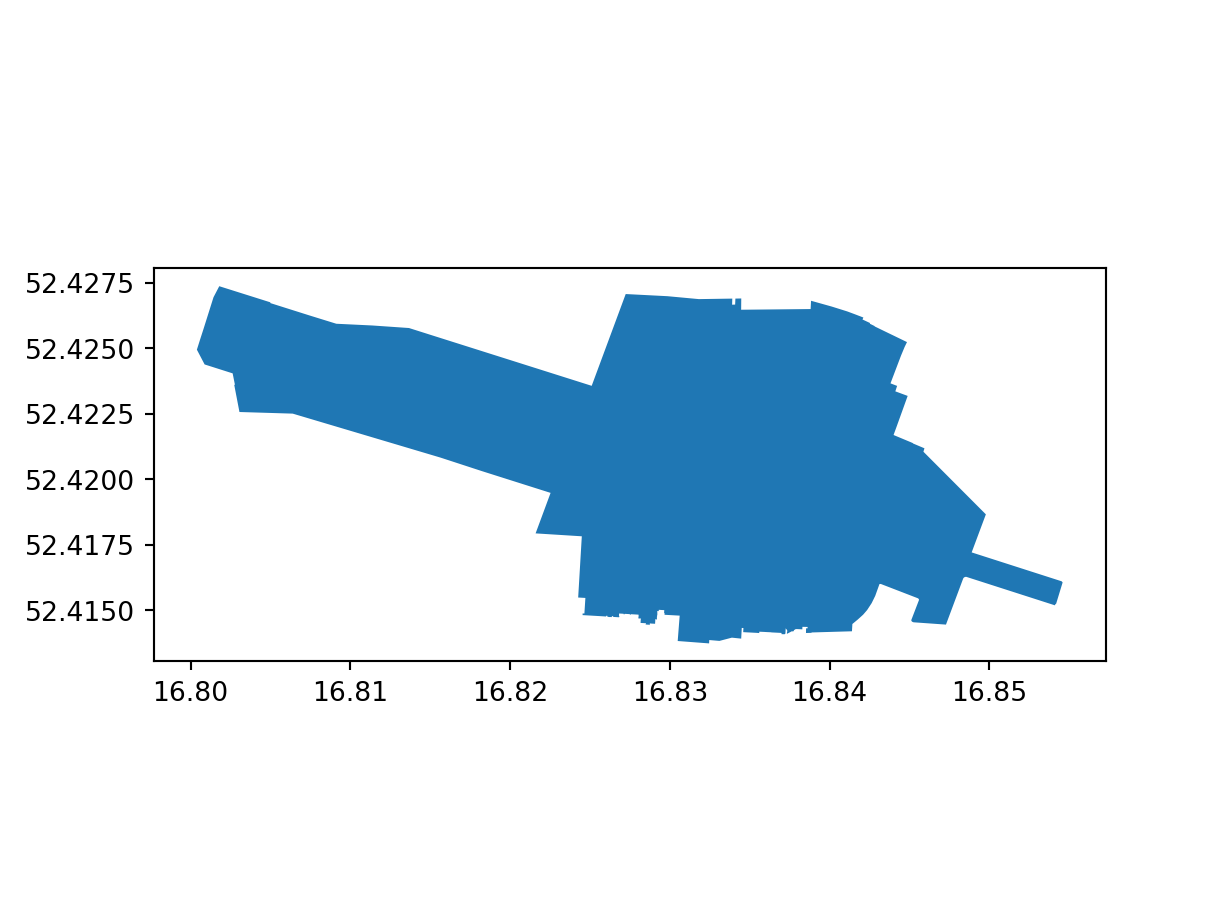``plot(pol)``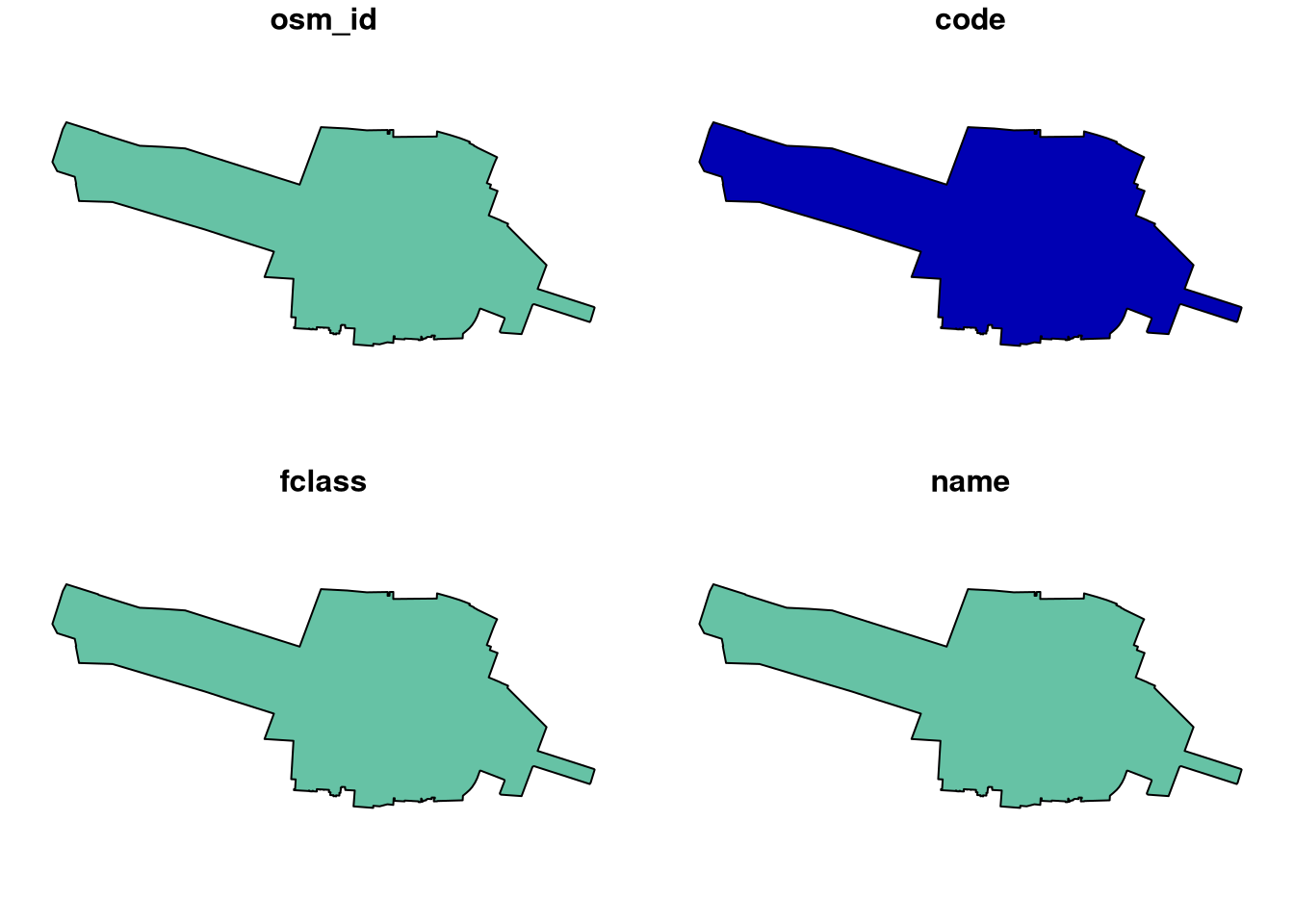The arguments needed to change the colour of the fill and border are different in R and Python, but the results are similar.

``pol.plot(color='none', edgecolor='black');``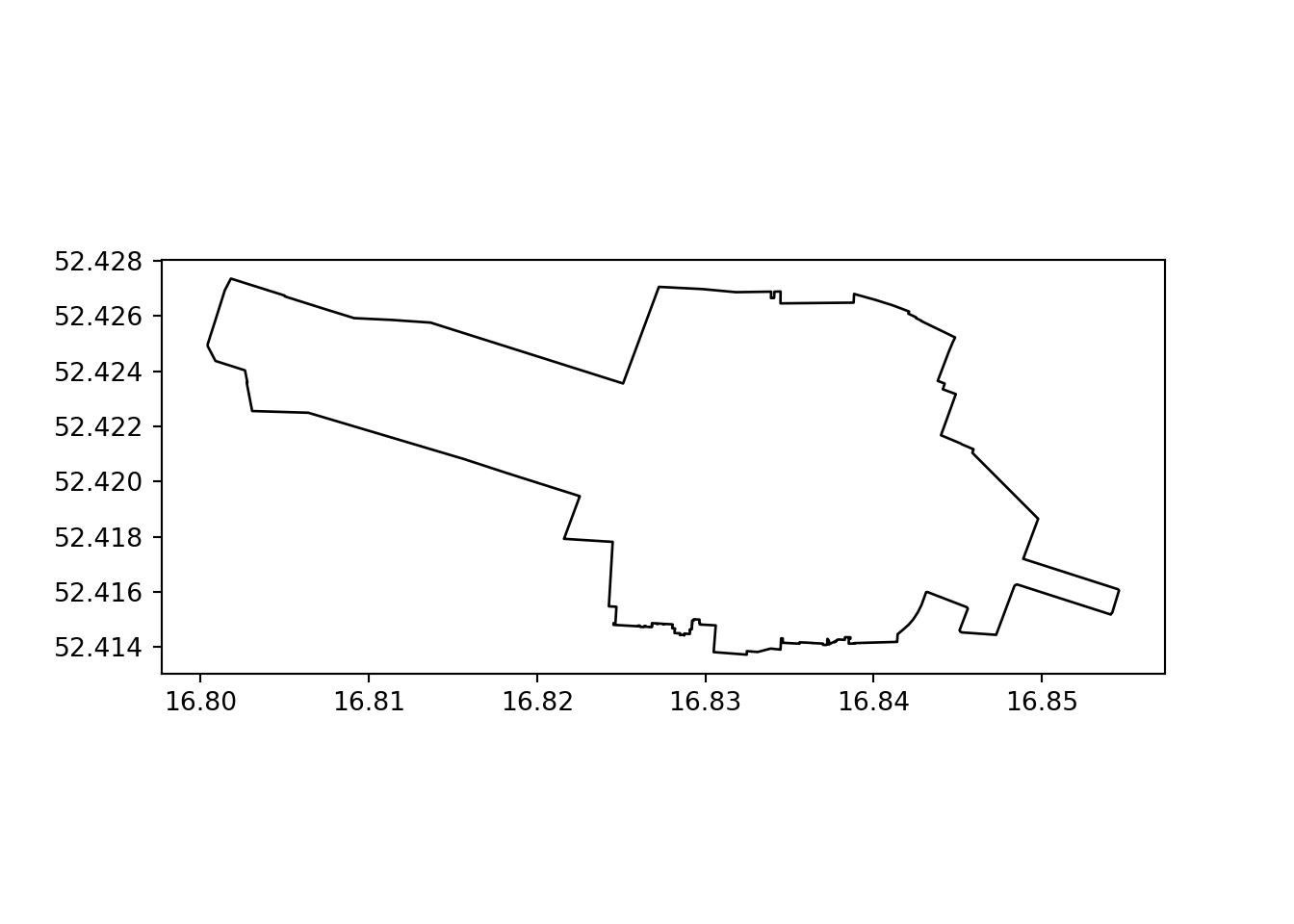``plot(st_geometry(pol), col = "white", border = "black")``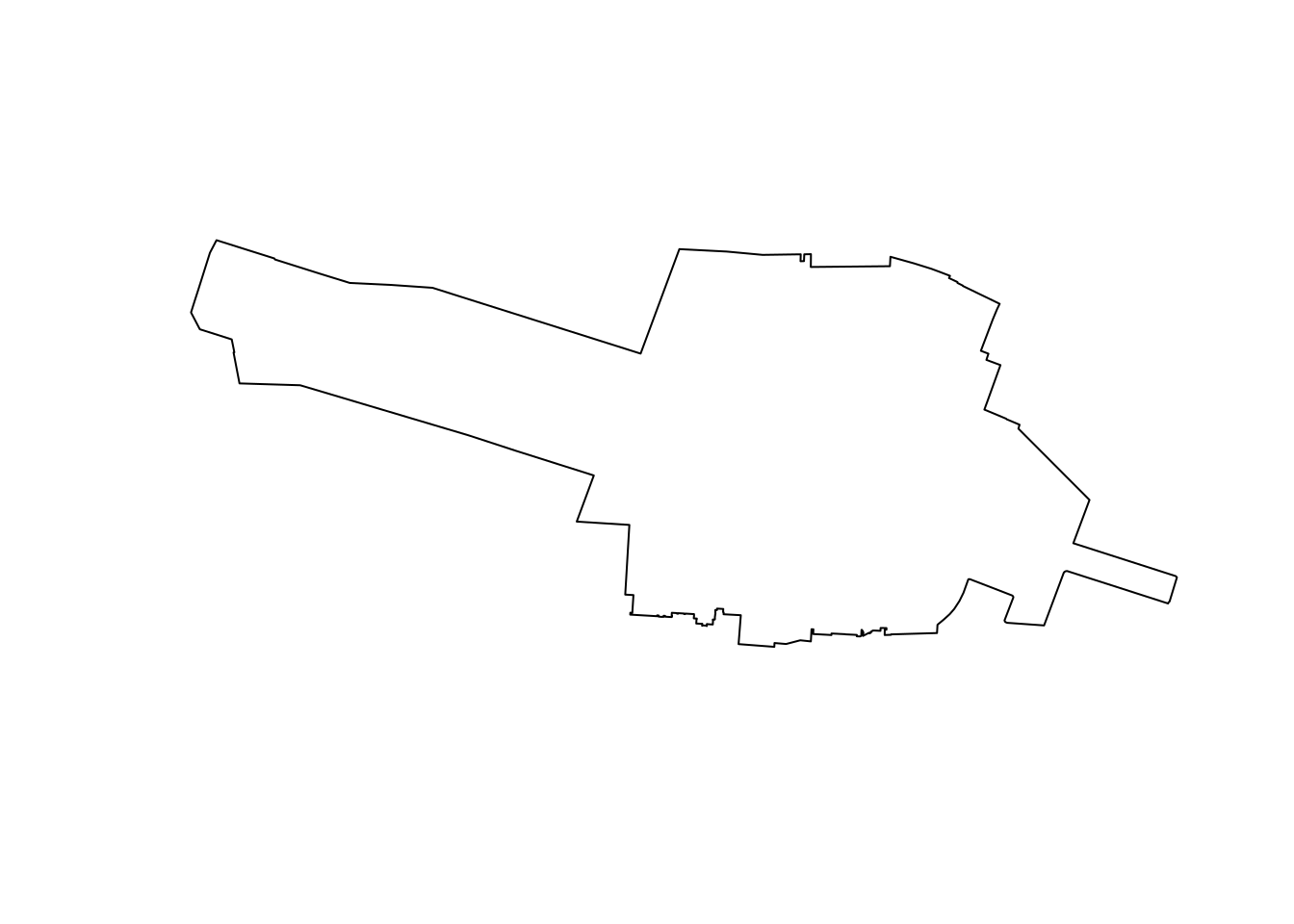## Creating geographic data frames from a CSV file

The following commands create a geographic data frame from a CSV file. Note that two steps—creating the geometry column and combining it with the original table, hereby combined into one complex expression—are needed to convert a `DataFrame` to a `GeoDataFrame` in Python, whereas in R the `sf::st_as_sf()` function can be used to convert a `data.frame` to a spatial data frame directly.

``````# Unzip the data.zip file:
with zipfile.ZipFile(f, 'r') as zip_ref:
zip_ref.extractall("data")
stops = gpd.GeoDataFrame(
stops.drop(columns=['stop_lon', 'stop_lat', 'stop_code']),
geometry = gpd.points_from_xy(stops.stop_lon, stops.stop_lat),
crs = 4326)
stops``````
``````      stop_id  ...                   geometry
0        2186  ...  POINT (17.04263 52.32684)
1         355  ...  POINT (16.86888 52.46234)
2        4204  ...  POINT (16.78629 52.47810)
3        3885  ...  POINT (16.72401 52.47590)
4         494  ...  POINT (16.93085 52.43616)
...       ...  ...                        ...
2916     2099  ...  POINT (16.65026 52.47006)
2917     3915  ...  POINT (16.98360 52.38233)
2918     3876  ...  POINT (16.52949 52.49770)
2919      594  ...  POINT (16.80900 52.43642)
2920     1190  ...  POINT (16.99819 52.44124)

[2921 rows x 4 columns]``````
``````stops = read_csv("data/gtfs/stops.txt") |>
select(-stop_code) |>
st_as_sf(coords = c("stop_lon", "stop_lat"), crs = "EPSG:4326")
stops``````
``````Simple feature collection with 2921 features and 3 fields
Geometry type: POINT
Dimension:     XY
Bounding box:  xmin: 16.48119 ymin: 52.15166 xmax: 17.27693 ymax: 52.58723
Geodetic CRS:  WGS 84
# A tibble: 2,921 × 4
stop_id stop_name              zone_id            geometry
*   <dbl> <chr>                  <chr>           <POINT [°]>
1    2186 Żerniki/Skrzyżowanie   B       (17.04263 52.32684)
2     355 Sucholeska             A       (16.86888 52.46234)
3    4204 Pawłowicka             A        (16.78629 52.4781)
4    3885 Kobylniki/Karolewska   B        (16.72401 52.4759)
5     494 Połabska               A       (16.93085 52.43616)
6    2040 Tarnowo Pdg/Karolewo I C       (16.68462 52.46915)
7    3736 Komorniki/Kryształowa  B        (16.78291 52.3478)
8    3932 Unii Lubelskiej        A        (16.9497 52.37239)
9    2795 Potasze/Jodłowa        C       (17.02994 52.52445)
10    3861 Miękowo/Stokrotkowa    C       (16.98954 52.49024)
# ℹ 2,911 more rows``````

## Plotting attributes and layers

The following commands plot the bus stops loaded in the previous step. Note that the tmap package is hereby used in R to create these more advanced plots, as it also supports interactive mapping (see below).

``stops.plot(markersize=1, column='zone_id', legend=True);``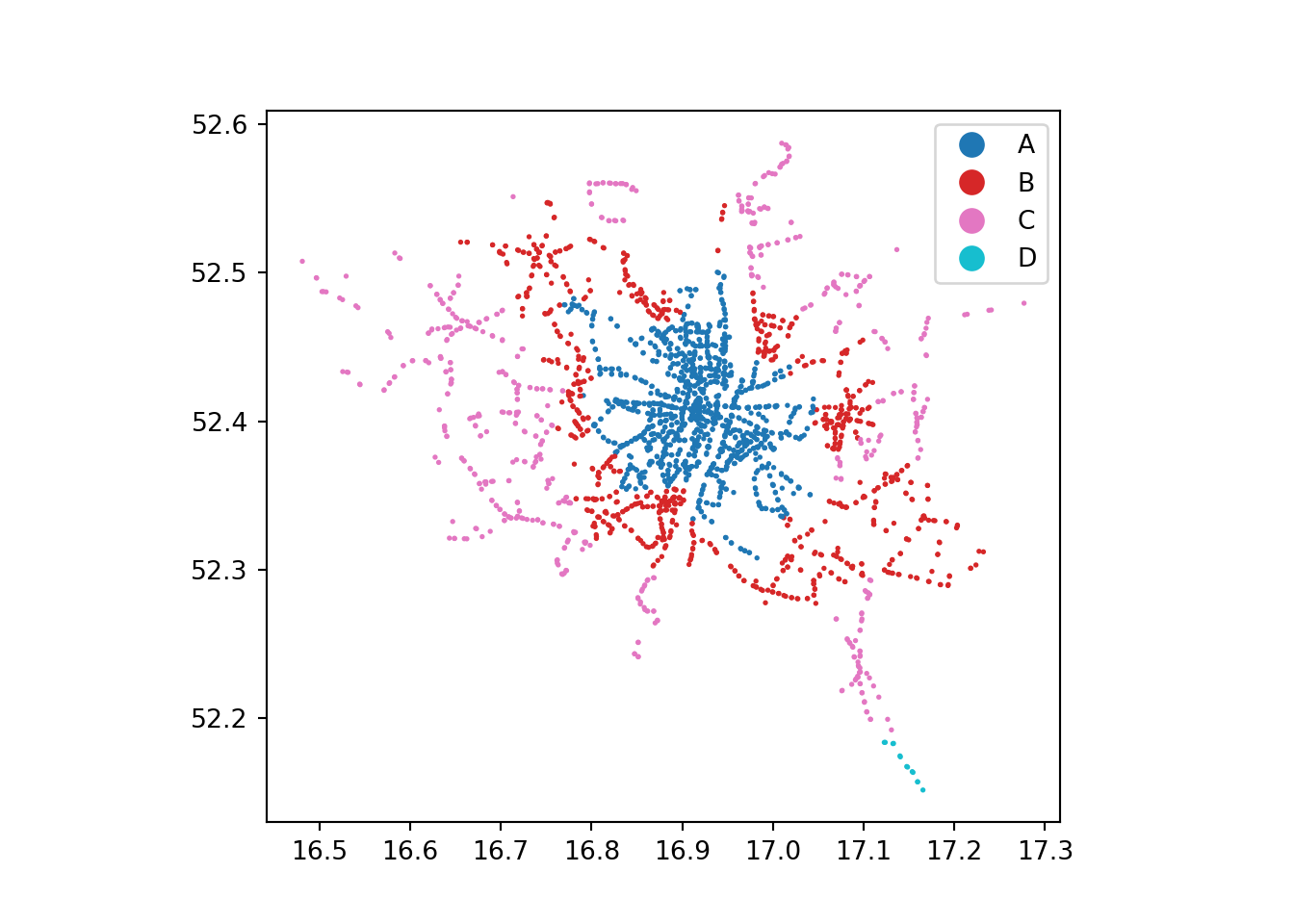``````tm_shape(stops) +
tm_symbols(size = 0.1, col = "zone_id")``````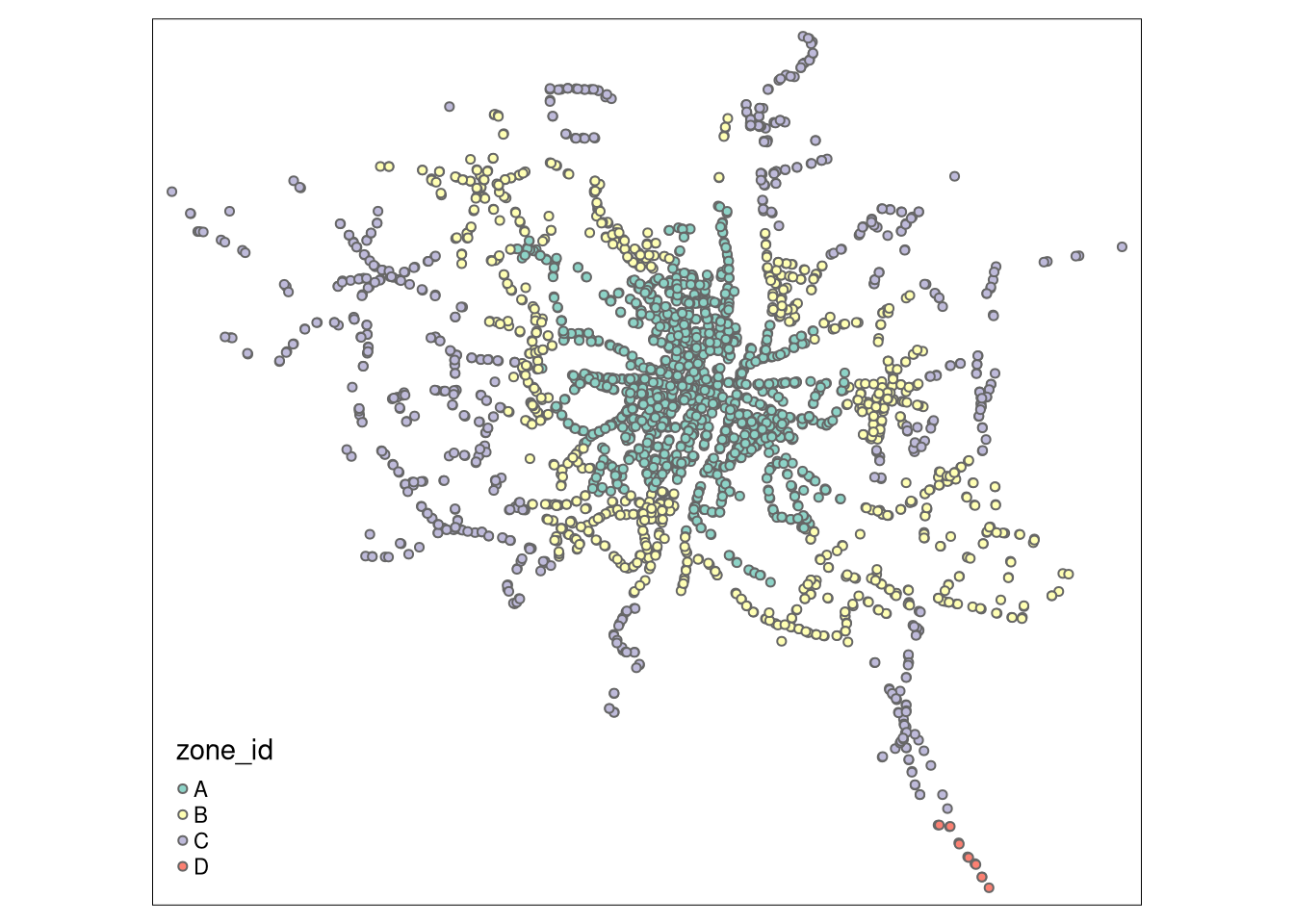We can add basic overlays in both languages as follows.

``````base = stops.plot(markersize=0.1)
poi.plot(ax=base, color='red');``````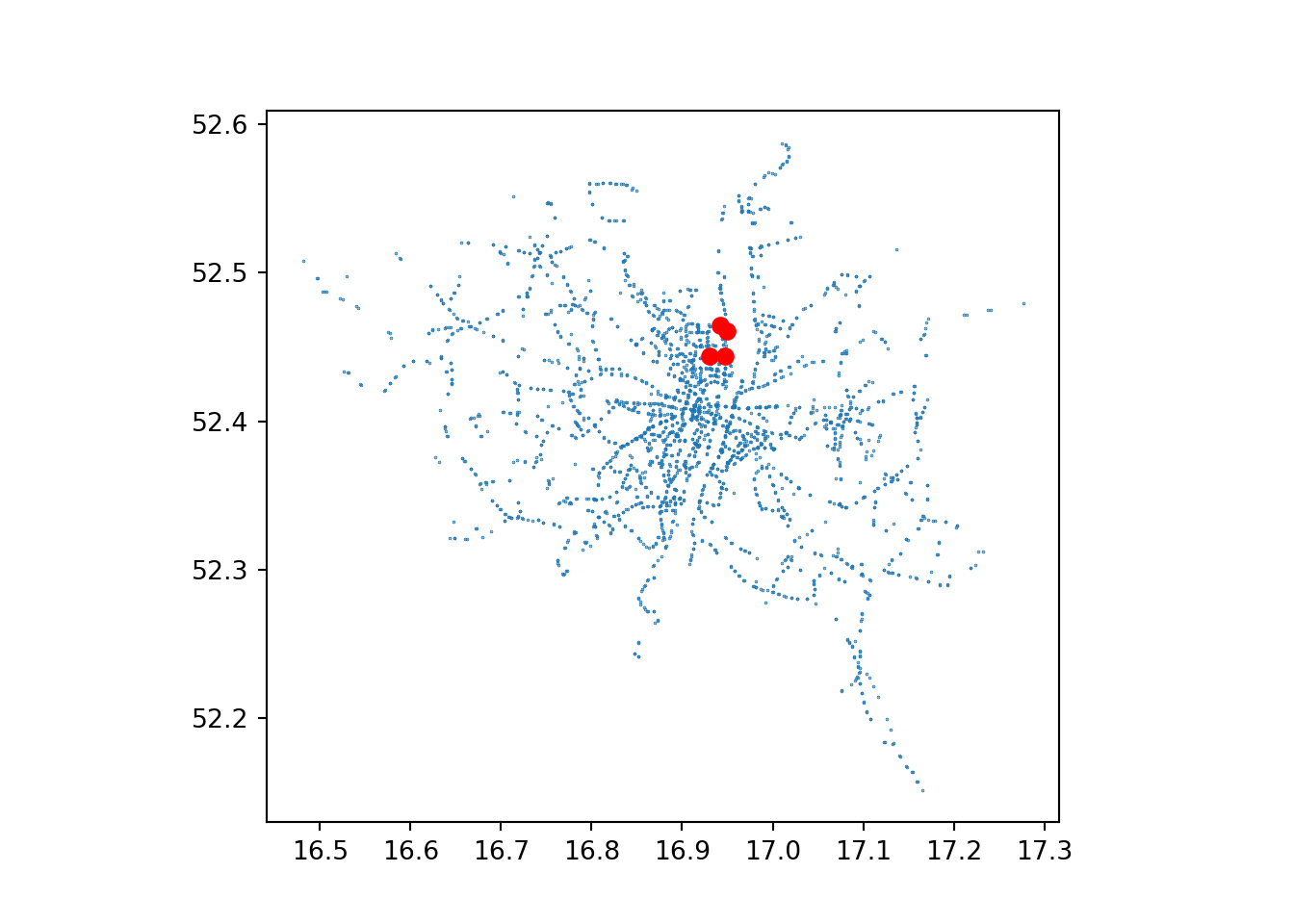``````plot(stops\$geometry, col = "grey", pch = 20, cex = 0.5)
plot(poi_sf\$geometry, col = "red", add = TRUE)``````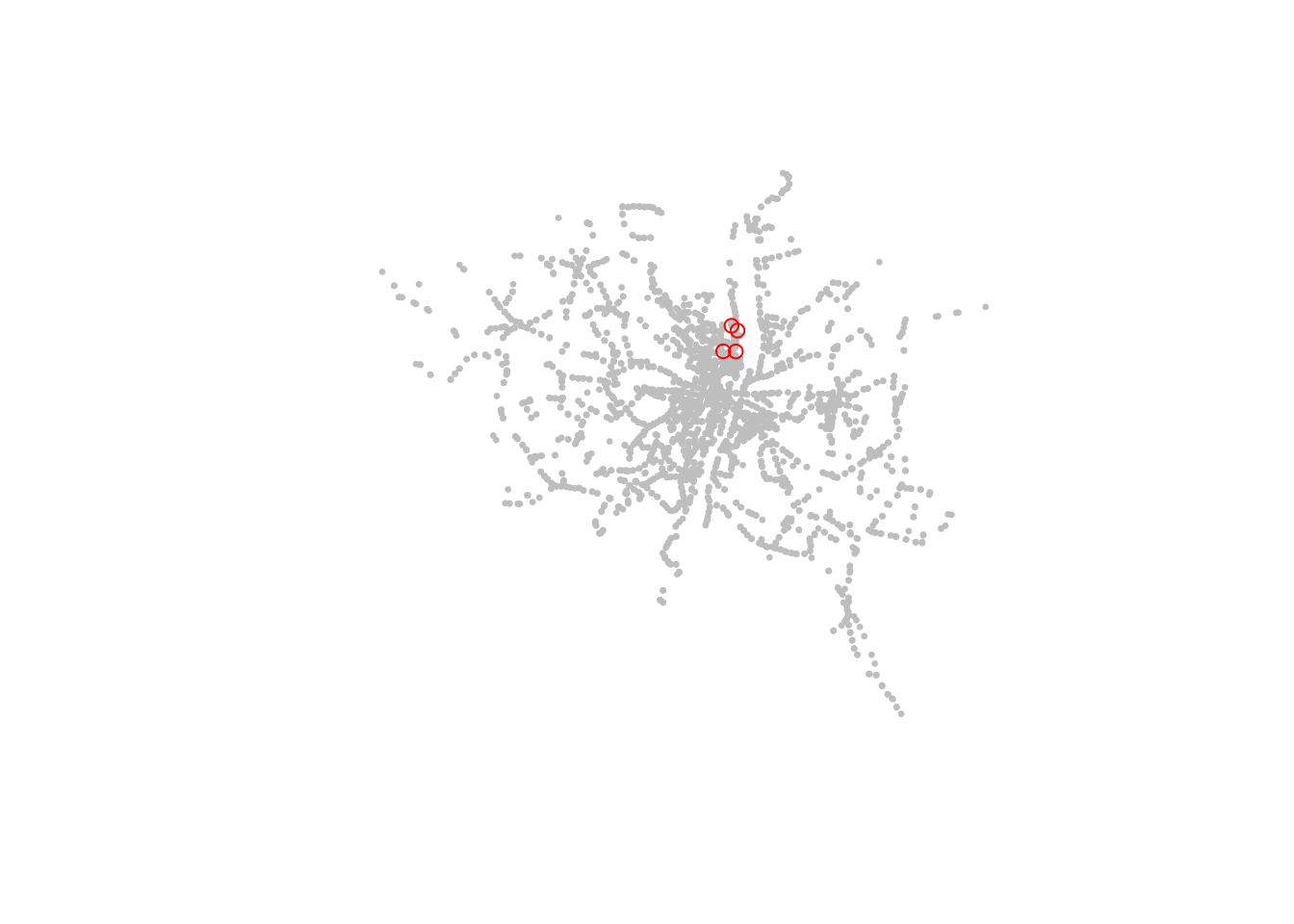## Interactive plots

The following commands create interactive plots, in Python and R respectively. The Python code requires the folium and mapclassify packages, which are not installed by default when you install geopandas. Note that with tmap, you can use the same code to create static and interactive plots, by changing the `tmap_mode()`.

``stops.explore(column='zone_id', legend=True, cmap='Dark2')``
Make this Notebook Trusted to load map: File -> Trust Notebook